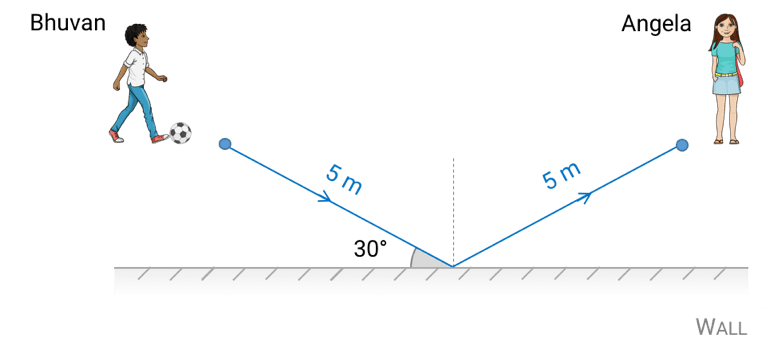Physics 09/02/2019

# Multi-topic questions in GCSE Physics

By KEVIN QUINN

Questions covering material from more than one topic (teachers call them synoptic questions) are designed to truly stretch your knowledge and understanding of the GCSE course. If you want to go in fighting 👊 and come out smiling 🙂… read on.

# 5-step method for multi-topic questions

Multi-topic questions are tough, so they will normally appear towards the end of the exam paper. These questions may be challenging, but they can be done by following a few simple steps.

Read the entire question carefully. Information that appears further down the page (or on the following page) may give you a clue as to how best to tackle an earlier part of the question.

### Step 2 – breathe

Take a moment to let your mind absorb the information which you’ve just read, and relax. You’ve watched My GCSE Science videos, completed the exam-style questions and tackled some past papers. You’re in a strong position – this what you’ve prepared for.

### Step 3 – highlight

Pay close attention to any key words and phrases which appear. Highlight them if you wish. Which topic(s) do they ‘belong’ to?

### Step 4 – equations

If you’ve been asked to calculate something, which equations from the topics which you’ve just identified might you be able to use? Simply writing down the correct equation(s) could get you a mark or two, depending on the mark scheme.

### Step 5 – attempt

Even if you’re not sure how to answer the entire question, attempt as much of it as you can. As well as maximising your score, correctly answering part of the question may give you a clue how to deal with the rest of it.

### Example

Bhuvan kicks a football along the ground towards a wall. The ball bounces off the wall and rolls towards Angela. As shown in the diagram below, the angle between the initial path of the ball and the wall is 30° and the total distance travelled by the ball is 10 m.a) The ball obeys the law of reflection as it strikes the wall.
State its angle of reflection from the wall. 

b) The mass of the ball is 500 g, and its initial speed is 4 m/s.
Show that its initial kinetic energy is 4 joules. 

c) One-tenth of the kinetic energy of the ball is transferred to other energy stores as it bounces off the wall. Calculate how long it takes for the ball to travel from Bhuvan to Angela. In answering this question, neglect all other losses of energy from the ball. 

### Prepare and solve

Before you attempt this question, take another look through the 5-step method shown above. Have you read the entire question carefully and highlighted any key words or phrases? Do you know which topics the question is testing, and which equations you might have to use?

Hopefully, you’ll have spotted that the law of reflection comes from the waves topic, and it states that ‘the angle of incidence is equal to the angle of reflection’. The angle of reflection (or incidence) is the angle between the reflected (or incident) ray and the normal, which means that the answer will be:

a) Angle of reflection = 90 – 30 = 60° 

The next part of this question is a straightforward ‘show that’ question, as long as you remember that the mass of the ball has to be converted to kilograms in the equation for the kinetic energy of an object.

b) Kinetic energy = ½ × mass × speed = ½ × 0.5 × 42 = 4 J  

The final part of the question requires more work, but a whole 5 marks are up for grabs. The answer to part a) isn’t needed here and we’ve already been given the initial kinetic energy of the ball in part b). So even if you’ve forgotten the law of reflection and had slipped up when calculating the ball’s initial kinetic energy, you could still answer the final part of this question in full. Good exam technique is to always attempt all parts of each question and pick up those marks!

Back to part c) of the question. The easiest way to perform this calculation is to split it into two parts – first, the time which the ball takes to get from Bhuvan to the wall, and second, the time which it takes to get from the wall to Angela. Because one-tenth of the kinetic energy of the ball is lost as it bounces, it must still have nine-tenths (or 90%) of its initial kinetic energy as it travels between the wall and Angela.

c) Time ball takes from Bhuvan to wall, t1= distance ÷ speed = 5 ÷ 4 = 1.25 s

Kinetic energy of ball after bouncing from wall = 0.9 × 4 = 3.6 J

Final speed of ball (after bouncing off wall):
Kinetic energy = ½ × mass × speed2
3.6 = ½ × 0.5 × speed2
Speed = 3.79 m/s

Time ball takes from wall to Angela, t2= distance ÷ speed = 5 ÷ 3.79 = 1.32 s

Time ball takes to get from Bhuvan to Angela = t1+ t2= 1.25 + 1.32 = 2.57 s

This is a challenging question, covering different aspects of the course. But if you follow our 5-step method of approaching the question thoughtfully and then break the question down into smaller parts then you can do it!

## One last thing

Well done on getting this far. Now take a quick break and then check through the specimen and past paper questions which are available on the exam board’s website.

Can you spot any multi-topic questions? Give them a go, using the 5-step method above, before checking your answers using the mark scheme.

Good luck! 👍

Kevin Quinn